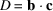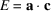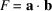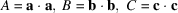International Tables for Crystallography (2006). Vol. A, ch. 9.2, pp. 750-755https://doi.org/10.1107/97809553602060000518

## Contents

• 9.2. Reduced bases  (pp. 750-755)
• 9.2.1. Introduction  (p. 750) | html | pdf |
• 9.2.2. Definition  (p. 750) | html | pdf |
• 9.2.3. Main conditions  (pp. 750-751) | html | pdf |
• 9.2.4. Special conditions  (pp. 751-754) | html | pdf |
• 9.2.5. Lattice characters  (pp. 754-755) | html | pdf |
• 9.2.6. Applications  (p. 755) | html | pdf |
• 9.2.6.1. Classification  (p. 755) | html | pdf |
• 9.2.6.2. Comparison of lattices  (p. 755) | html | pdf |
• References | html | pdf |
• Figures
• Fig. 9.2.4.1. The net of lattice points in the plane of the reduced basis vectors a and b ; OBAD is a primitive mesh  (p. 751) | html | pdf |
• Fig. 9.2.4.2. The effect of the special conditions  (p. 752) | html | pdf |
• Fig. 9.2.4.3. The effect of the special conditions  (p. 752) | html | pdf |
• Fig. 9.2.4.4. The effect of the special conditions  (p. 752) | html | pdf |
• Fig. 9.2.4.5. The effect of the special conditions  (p. 752) | html | pdf |
• Tables
• Table 9.2.5.1. The parameters,andof the 44 lattice characters ()  (p. 753) | html | pdf |
• Table 9.2.5.2. Lattice characters described by relations between conventional cell parameters  (p. 754) | html | pdf |